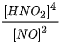# Problem: Give the individual reaction orders for all substances and the overall reaction order from this rate law: rate = k [HNO2]4[NO]2A. order of HNO2 = ?B. order of NO = ?C. overall order = ?D. By what factor does the rate change if the concentration of HNO2 is doubled = ?E. By what factor does the rate change if the concentration of NO is doubled = ?F. By what factor does the rate change if the concentration of HNO2 is tripled and the concentration of NO is halved = ?

###### FREE Expert Solution

Recall that the rate law only focuses on the reactant concentrations and has a general form of:

k = rate constant
A & B = reactants
x & y = reactant orders

Given:

90% (363 ratings)###### Problem Details

Give the individual reaction orders for all substances and the overall reaction order from this rate law: rate = k[HNO2]4[NO]2

A. order of HNO2 = ?

B. order of NO = ?

C. overall order = ?

D. By what factor does the rate change if the concentration of HNO2 is doubled = ?

E. By what factor does the rate change if the concentration of NO is doubled = ?

F. By what factor does the rate change if the concentration of HNO2 is tripled and the concentration of NO is halved = ?